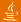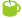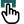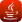### Found in titles:

1.DMelt: org.apache.poi.ss.formula.functions.Finance [99%] (Java API)
2.Jython Example: finance_timeseries.py [100%] A plot with time series.
3.Wiki: DMelt:Finance/Trade Derivatives [94%] (in title)
4.Wiki: DMelt:Finance/Financial Computations [94%] (in title)
5.Wiki: DMelt:Finance/Financial Charts [94%] (in title)
6.Wiki: DMelt:Finance/Market Prediction [94%] (in title)
7.Wiki: DMelt:Finance/Time Series [94%] (in title)
8.Jython Example: finance2.py [70%] Calculating compound interest.
9.Jython Example: market_predict_evaluate.py [58%] Market predictions using NN
10.Jython Example: finance1.py [58%] Calculates equity option values with a number of methods
11.Jython Example: time_series2.py [58%] Read time series and plot it
12.Jython Example: timeseries_chart.py [58%] A timeseries based on jFreeChart with moving average
13.Java Example: ForecastingChartDemo.java [58%] Time series observations with different forecasting models
14.Java Example: ExponentialSmoothingChartDemo.java [58%] Time series observations with smoothing forecasts
15.Jython Example: market_predict_train.py [47%] Market predictions using NN (NN training)
16.Jython Example: time_series1.py [47%] Read time series and export to alternative formats (ASCII, Gauss, Matlab, Excel)
17.Jython Example: time_series3.py [47%] Read time series and plot using castom X-lables (months)
18.Jython Example: market_predict_getdata.py [47%] Market predictions using NNi (fetch data)
19.Jython Example: candlestick_chart.py [47%] A candlestick chart used to describe price movements of a security, derivative, or currency.
20.Jython Example: nasdaq_values.py [47%] Plot values of NASDAQ companies using CSV file
Searching 62556 articles took 14 ms